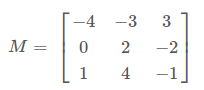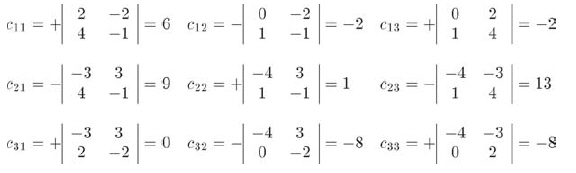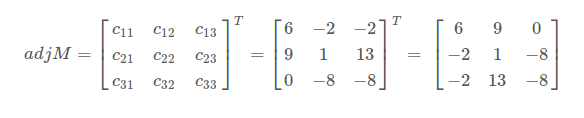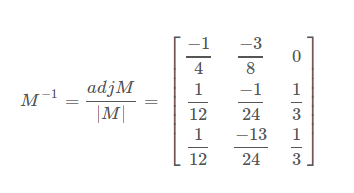矩阵的求逆

逆的几何解释

$$(\vec{v}M)M^{-1}=\vec{v}(MM^{-1})=\vec{v}I=\vec{v}$$

标准伴随矩阵M的标准伴随矩阵是代数余子式矩阵的转置:求逆

$$M^{-1}=\frac {adjM}{|M|}$$